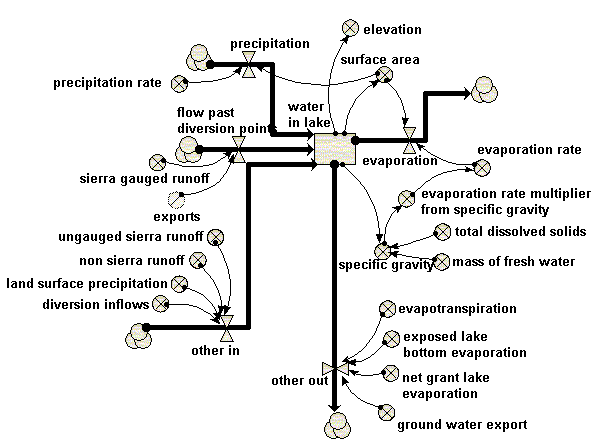# Model entry

Mono Lake, California: 3rd model - Model catalogue - Simulistics.com

 Search Simulistics Model catalogue Listed by keyword Listed by ID Listed by title Listed by date added

# Mono Lake, California: 3rd model

Model : mono3
Simile version : 3.1+
Keywords : Lake model ; Mono Lake, California ; Lumped model ; Water ;

## Description

Mono Lake is a lake in the Sierra Nevada, California, that was used to supply water to Los Angeles, and is also of considerable ecological importance. This is the first of a series of models developed as a teaching exrcise to explore the effects of different policies for water extraction.

This model uses a single compartment to represent the amount of water in the lake, with inflows representing precipitation, flow in past diversion points and other sources; and flows out for evaporation and other losses (extraction).

This model differs from the first in that the surface area of the lake now depends on the volume of water in the lake, rather than being assumed to be constant.

Source: Andrew Ford (1999): Modelling the Environment, p.42

dW/dt = P+F+O-E-X
P = p*sa
E = e*sa
F = r-ex

sa = graph(W) Where:
W = water in lake
P = precipitation
F = fl = ow past diversion points
O = other flow in
E = evaporation
X = extraction
p = precipitation rate
sa = surface area
e = evaporation rate
r = sierra gauged runoff
ex = export

## Diagram## Equations

```Compartments:
water in lake
Initial value = 2228
Comments: Amount of water in lake, KAF (thousand acre-feet)

Flows:
evaporation = surface_area*evaporation_rate

flow past diversion points = sierra_gauged_runoff-export

other in = ungauged_sierra_runoff+non_sierra_runoff+land_surface_precipitation+diversion_inflows

other out = evapotranspiration+exposed_lake_bottom_evaporation+net_grant_lake_evaporation+ground_water_export

precipitation = surface_area*precipitation_rate

Variables:
elevation = graph(water_in_lake)

evaporation rate = 3.75*evaporation_rate_multiplier_from_specific_gravity

evaporation rate multiplier from specific gravity = graph(specific_gravity)

specific gravity = (water_in_lake*mass_of_fresh_water+total_dissolved_solids)/(water_in_lake*mass_of_fresh_water)

surface area = graph(water_in_lake)

Parameters:
evapotranspiration = 13

export = 100

exposed lake bottom evaporation = 12

ground water export = 7.3

land surface precipitation = 9

mass of fresh water = 1.359
Comments: million tons per thousand acre-feet

net grant lake evaporation = 1.3

non sierra runoff = 20

precipitation rate = 0.667

sierra gauged runoff = 150

total dissolved solids = 230
```

## Results

||

## References

Ford, A (1999) Modeling the Environment.
Island Press. p.42